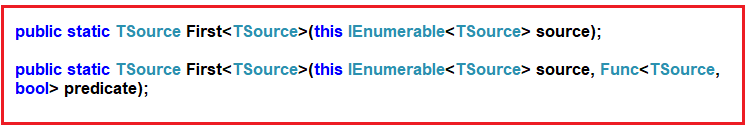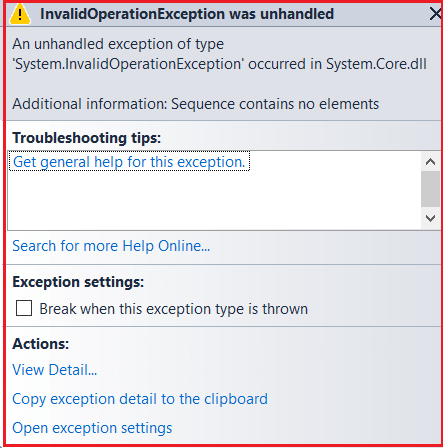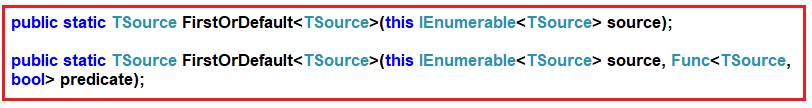# First and FirstOrDefault Methods in Linq

## First and FirstOrDefault Methods in Linq

In this article, I am going to discuss the First and FirstOrDefault Methods in Linq with some examples. Please read our previous article before proceeding to this article, where we discussed the ElementAt and ElementAtOrDefault method in Linq.

##### First Method in Linq:

The Linq First Method is used to return the first element from a data source. If the data source is empty, then this method will throw an exception. There are two overloaded versions available for this method as shown below.As you can see, the first overloaded version does not take any parameter and it simply returns the first element from the data source. The second overloaded version takes a predicate as a parameter using which we can specify a condition and then it will return the first element which satisfied the specified condition.

##### Example1: Return the first element from a data source.

In the below example, we have created one data source which contains integer numbers from 1 to 10. So, our requirement is to fetch the first element from the data source i.e. in the output we want to display the value 1 as it is the first element in the data source.

```using System.Linq;
using System;
using System.Collections.Generic;
namespace LINQDemo
{
class Program
{
static void Main(string[] args)
{
List<int> numbers = new List<int>() { 1, 2, 3, 4, 5, 6, 7, 8, 9, 10 };
int MethodSyntax = numbers.First();
Console.WriteLine(MethodSyntax);

}
}
}
```

Now, run the application and it should display 1 in the output window as expected.

##### Example2: Fetch the first element from the data source which is divisible by 2.

Here we need to use the second overloaded version of the First method using which we can specify our condition. The following program uses the second overloaded version to return the first element from the data source which is divisible by 2.

```using System.Linq;
using System;
using System.Collections.Generic;
namespace LINQDemo
{
class Program
{
static void Main(string[] args)
{
List<int> numbers = new List<int>() { 1, 2, 3, 4, 5, 6, 7, 8, 9, 10 };
int MethodSyntax = numbers.First(num => num % 2 == 0);
Console.WriteLine(MethodSyntax);

}
}
}
```

Output: 2

This is because 2 is the first element in the data source which is divisible by 2.

##### Example3: InvalidOperationException

Whenever the data source is empty or if the specified condition does not return any data, then we will get the InvalidOperationException as shown in the below example.

```using System.Linq;
using System;
using System.Collections.Generic;
namespace LINQDemo
{
class Program
{
static void Main(string[] args)
{
//Empty Data Source
List<int> numbersEmpty = new List<int>() { };
int MethodSyntax1 = numbersEmpty.First();

//Specified condition doesnot return any element
List<int> numbers = new List<int>() { 1, 2, 3, 4, 5, 6, 7, 8, 9, 10 };
int MethodSyntax2 = numbers.First(num => num > 50);

}
}
}
```

Now if you run the application, you will get the following exception.If you don’t want that Invalid Operation Exception, instead you want a default value based on the data type then you need to use the FirstOrDefault method.

Note: For the reference types, the default is NULL and for the value types, the default depends on the actual type expected.

##### FirstOrDefault Method in Linq:

The Linq FirstOrDefault method exactly does the same thing as the First method except that this method does not throw Invalid Operation Exception instead it returns the default value based on the data type of the element. Like the First method, there are also two overloaded versions available for the FirstOrDefault method as shown below.##### Example4: Fetch the element from the data source using FirstOrDefault.
```using System.Linq;
using System;
using System.Collections.Generic;
namespace LINQDemo
{
class Program
{
static void Main(string[] args)
{
List<int> numbers = new List<int>() { 1, 2, 3, 4, 5, 6, 7, 8, 9, 10 };
int MethodSyntax = numbers.FirstOrDefault();
Console.WriteLine(MethodSyntax);

}
}
}
```

Output: 1

##### Example5: Fetch the element from the data source which is greater than 5.
```using System.Linq;
using System;
using System.Collections.Generic;
namespace LINQDemo
{
class Program
{
static void Main(string[] args)
{
List<int> numbers = new List<int>() { 1, 2, 3, 4, 5, 6, 7, 8, 9, 10 };
int MethodSyntax = numbers.FirstOrDefault(num => num > 5);
Console.WriteLine(MethodSyntax);

}
}
}
```

Output: 6

##### Example6:

In the below example, we have two data sources. The first data source does not contain any item and here we are trying the fetch the first element. The second data source contains 10 elements from the number 1 to 10 and from this data source we are trying to retrieve the first element which is greater than 50.

```using System.Linq;
using System;
using System.Collections.Generic;
namespace LINQDemo
{
class Program
{
static void Main(string[] args)
{
//Empty Data Source
List<int> numbersEmpty = new List<int>() { };
int MethodSyntax1 = numbersEmpty.FirstOrDefault();
Console.WriteLine(MethodSyntax1);

//Specified condition doesnot return any element
List<int> numbers = new List<int>() { 1, 2, 3, 4, 5, 6, 7, 8, 9, 10 };
int MethodSyntax2 = numbers.FirstOrDefault(num => num > 50);
Console.WriteLine(MethodSyntax2);

}
}
}
```

Here it will print the values as 0 and 0. This is because the data source contains integers. And the default for integer is 0.

##### Example7: First and FirstOrDefault method Using Query Syntax

There is no such operator called First or FirstOrDefault is available to write the query syntax, If you want then you can combine both the method syntax and query syntax to write the code as shown below.

```using System.Linq;
using System;
using System.Collections.Generic;
namespace LINQDemo
{
class Program
{
static void Main(string[] args)
{
List<int> numbers = new List<int>() { 1, 2, 3, 4, 5, 6, 7, 8, 9, 10 };

int QuerySyntax1 = (from num in numbers
select num).First();

int QuerySyntax2 = (from num in numbers
select num).FirstOrDefault();

Console.WriteLine(QuerySyntax1);
Console.WriteLine(QuerySyntax2);